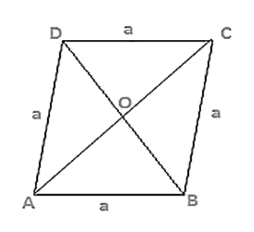# A rhombus sheet, whose perimeter is 32 m and whose diagonal is 10 m long, is painted on both the sides at the rate of Rs 5 per meter square. Find the cost of painting.

Question:

A rhombus sheet, whose perimeter is 32 m and whose diagonal is 10 m long, is painted on both the sides at the rate of Rs 5 per meter square. Find the cost of painting.

Solution:

Given that,

Perimeter of a rhombus = 32 m

We know that,

Perimeter of a rhombus = 4 × side

4 × side = 32 m

4 × a = 32 m

a = 8 m

Let AC = 10 m

OA = 12 × AC

OA = 12 × 10

OA = 5 m

By using Pythagoras theorem

$O B^{2}=A B^{2}-O A^{2}$

$O B^{2}=8^{2}-5^{2}$

$O B=\sqrt{39} m$

$B D=2 \times O B$

$B D=2 \sqrt{39} m$

Area of the sheet $=1 / 2 \times \mathrm{BD} \times \mathrm{AC}$

Area of the sheet $=1 / 2 \times 2 \sqrt{39} \times 10$

Therefore, cost of printing on both sides at the rate of Rs. 5 per $\mathrm{m}^{2}$

$=$ Rs $2 \times 10 \sqrt{39} \times 5$

$=$ Rs. 625